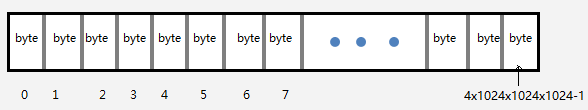# C语言指针详解

## 指针是什么？## 为什么程序中的数据会有自己的地址？#include <stdio.h>

int main(void)
{
char ch = 'a';
int  num = 97;
printf("ch 的地址:%p\n",&ch);   //ch 的地址:0028FF47
printf("num的地址:%p\n",&num);  //num的地址:0028FF40
return 0;
}## 变量和内存

为了简单起见，这里就用上面例子中的  int num = 97 这个局部变量来分析变量在内存中的存储模型。1、内存的数据
内存的数据就是变量的值对应的二进制，一切都是二进制。97的二进制是 : 00000000 00000000 00000000 0110000 , 但使用的小端模式存储时，低位数据存放在低地址，所以图中画的时候是倒过来的。

2、内存数据的类型
内存的数据类型决定了这个数据占用的字节数，以及计算机将如何解释这些字节。num的类型是int，因此将被解释为 一个整数。

3、内存数据的名称
内存的名称就是变量名。实质上，内存数据都是以地址来标识的，根本没有内存的名称这个说法，这只是高级语言提供的抽象机制 ，方便我们操作内存数据。而且在C语言中，并不是所有的内存数据都有名称，例如使用malloc申请的堆内存就没有。

4、内存数据的地址
如果一个类型占用的字节数大于1，则其变量的地址就是地址值最小的那个字节的地址。因此num的地址是 0028FF40。 内存的地址用于标识这个内存块。

5、内存数据的生命周期
num是main函数中的局部变量，因此当main函数被启动时，它被分配于栈内存上，当main执行结束时，消亡。

无论以后计算机硬件如何发展，内存容量都是有限的，因此清楚理解程序中每一个程序数据的生命周期是非常重要的。
我会在以后的文章中再对C语言的内存管理做出介绍，敬请期待。

## 指针变量 和 指向关系C语言中，定义变量时，在变量名 前 写一个 * 星号，这个变量就变成了对应变量类型的指针变量。必要时要加( ) 来避免优先级的问题。

int a ; //int类型变量 a
int *a ; //int* 变量a
int arr; //arr是包含3个int元素的数组
int (* arr ); //arr是一个指向包含3个int元素的数组的指针变量

//-----------------各种类型的指针------------------------------

int* p_int; //指向int类型变量的指针

double* p_double; //指向idouble类型变量的指针

struct Student *p_struct; //结构体类型的指针

int(*p_func)(int,int); //指向返回类型为int，有2个int形参的函数的指针

int(*p_arr); //指向含有3个int元素的数组的指针

int** p_pointer; //指向 一个整形变量指针的指针

int add(int a , int b)
{
return a + b;
}

int main(void)
{
int num = 97;
float score = 10.00F;
int arr = {1,2,3};

//-----------------------

int* p_num = &num;
float* p_score = &score;
int (*p_arr) = &arr;
return 0;
}

• 数组名的值就是这个数组的第一个元素的地址。
• 函数名的值就是这个函数的地址。
• 字符串字面值常量作为右值时，就是这个字符串对应的字符数组的名称,也就是这个字符串在内存中的地址。
int add(int a , int b){
return a + b;
}
int main(void)
{
int arr = {1,2,3};
//-----------------------
int* p_first = arr;
const char* msg = "Hello world";
return 0;
}

int main(void)
{
int age = 19;
int*p_age = &age;
*p_age  = 20;  //通过指针修改指向的内存数据

printf("age = %d\n",*p_age);   //通过指针读取指向的内存数据
printf("age = %d\n",age);

return 0;
}

int* p1  = & num;
int* p3 = p1;

//通过指针 p1 、 p3 都可以对内存数据 num 进行读写，如果2个函数分别使用了p1 和p3，那么这2个函数就共享了数据num。#ifdef __cplusplus
#define NULL    0
#else
#define NULL    ((void *)0)
#endif

void opp()
{
int*p = NULL;
*p = 10;      //Oops! 不能对NULL解地址
}

void foo()
{
int*p;
*p = 10;      //Oops! 不能对一个未知的地址解地址
}

void bar()
{
int*p = (int*)1000;
*p =10;      //Oops!   不能对一个可能不属于本程序的内存的地址的指针解地址
}

## 指针的2个重要属性

int main(void)
{
int num = 97;
int *p1  = &num;
char* p2 = (char*)(&num);

printf("%d\n",*p1);    //输出  97
putchar(*p2);          //输出  a
return 0;
}

*p1  :  将从地址0028FF40 开始解析，因为p1是int类型指针，int占4字节，因此向后连续取4个字节，并将这4个字节的二进制数据解析为一个整数 97。
*p2  :  将从地址0028FF40 开始解析，因为p2是char类型指针，char占1字节，因此向后连续取1个字节，并将这1个字节的二进制数据解析为一个字符，即'a'。

void*类型指针

## 结构体和指针

typedef struct
{
char name;
int age;
float score;
}Student;

int main(void)
{
Student stu = {"Bob" , 19, 98.0};
Student*ps = &stu;

ps->age = 20;
ps->score = 99.0;
printf("name:%s age:%d\n",ps->name,ps->age);
return 0;
}

## 数组和指针

1、数组名作为右值的时候，就是第一个元素的地址。
int main(void)
{
int arr = {1,2,3};

int*p_first = arr;
printf("%d\n",*p_first);  //1
return 0;
}

2、指向数组元素的指针 支持 递增 递减 运算。（实质上所有指针都支持递增递减 运算 ，但只有在数组中使用才是有意义的）

int main(void)
{
int arr = {1,2,3};

int*p = arr;
for(;p!=arr+3;p++){
printf("%d\n",*p);
}
return 0;
}
3、p= p+1 意思是，让p指向原来指向的内存块的下一个相邻的相同类型的内存块。
同一个数组中，元素的指针之间可以做减法运算，此时，指针之差等于下标之差。

4、p[n]    == *(p+n)
p[n][m]  == *(  *(p+n)+ m )

5、当对数组名使用sizeof时，返回的是整个数组占用的内存字节数。当把数组名赋值给一个指针后，再对指针使用sizeof运算符，返回的是指针的大小。

int main(void)
{
int arr = {1,2,3};

int*p = arr;
printf("sizeof(arr)=%d\n",sizeof(arr));  //sizeof(arr)=12
printf("sizeof(p)=%d\n",sizeof(p));   //sizeof(p)=4

return 0;
}

## 函数和指针

C语言中，实参传递给形参，是按值传递的，也就是说，函数中的形参是实参的拷贝份，形参和实参只是在值上面一样，而不是同一个内存数据对象。这就意味着：这种数据传递是单向的，即从调用者传递给被调函数，而被调函数无法修改传递的参数达到回传的效果。
void change(int a)
{
a++;      //在函数中改变的只是这个函数的局部变量a，而随着函数执行结束，a被销毁。age还是原来的age，纹丝不动。
}
int main(void)
{
int age = 19;
change(age);
printf("age = %d\n",age);   // age = 19
return 0;
}

void change(int* pa)
{
(*pa)++;   //因为传递的是age的地址，因此pa指向内存数据age。当在函数中对指针pa解地址时，
//会直接去内存中找到age这个数据，然后把它增1。
}
int main(void)
{
int age = 19;
change(&age);
printf("age = %d\n",age);   // age = 20
return 0;
}

#include<stdio.h>
void swap_ok(int*pa,int*pb);

int main()
{
int a = 5;
int b = 3;
swap_ok(&a,&b);      //OK
return 0;
}

//错误的写法
{
int t;
t=a;
a=b;
b=t;
}

//正确的写法：通过指针
void swap_ok(int*pa,int*pb)
{
int t;
t=*pa;
*pa=*pb;
*pb=t;
}typedef struct
{
char name;
int age;
float score;
}Student;

//打印Student变量信息
void show(const Student * ps)
{
printf("name:%s , age:%d , score:%.2f\n",ps->name,ps->age,ps->score);
}

C语言中，函数名作为右值时，就是这个函数的指针。

void echo(const char *msg)
{
printf("%s",msg);
}
int main(void)
{
void(*p)(const char*) = echo;   //函数指针变量指向echo这个函数

p("Hello ");      //通过函数的指针p调用函数，等价于echo("Hello ")
echo("World\n");
return 0;
}

## const 和 指针

const到底修饰谁？谁才是不变的？

int main()
{
int a = 1;

int const *p1 = &a;        //const后面是*p1，实质是数据a，则修饰*p1，通过p1不能修改a的值
const int*p2 =  &a;        //const后面是int类型，则跳过int ，修饰*p2， 效果同上

int* const p3 = NULL;      //const后面是数据p3。也就是指针p3本身是const .

const int* const p4 = &a;  // 通过p4不能改变a 的值，同时p4本身也是 const
int const* const p5 = &a;  //效果同上

return 0;

}

typedef int* pint_t;  //将 int* 类型 包装为 pint_t,则pint_t 现在是一个完整的原子类型

int main()
{

int a  = 1;
const pint_t p1 = &a;  //同样，const跳过类型pint_t，修饰p1，指针p1本身是const
pint_t const p2 = &a;  //const 直接修饰p，同上

return 0;

}

## 深拷贝和浅拷贝## 附加知识

1) Little-Endian就是低字节排放在内存的低地址端，高字节排放在内存的高地址端。个人PC常用，Intel X86处理器是小端模式。
2) B i g-Endian就是高字节排放在内存的低地址端，低字节排放在内存的高地址端。

short a = 1;//测试机器使用的是否为小端模式。是，则返回true，否则返回false//这个方法判别的依据就是：C语言中一个对象的地址就是这个对象占用的字节中，地址值最小的那个字节的地址。
bool isSmallIndain()
{
unsigned int val = 'A';
unsigned char* p = (unsigned char*)&val;  //C/C++：对于多字节数据，取地址是取的数据对象的第一个字节的地址，也就是数据的低地址

return *p == 'A';
}
`

posted @ 2017-08-31 20:41  lulipro  阅读(...)  评论(...编辑  收藏